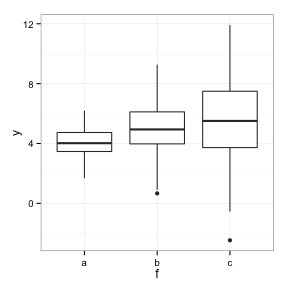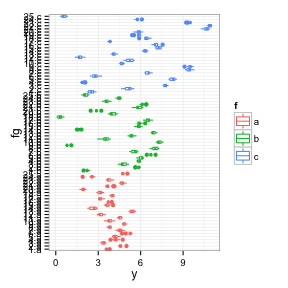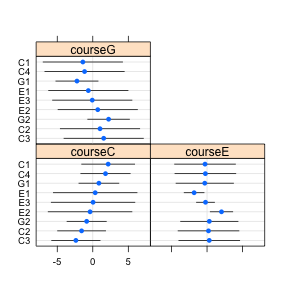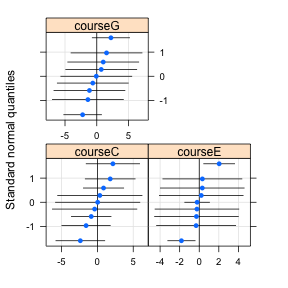# Factor-specific variances in R

Under construction!

A topic that has come up several times on the R list and elsewhere: (how) can one allow different variances among random effects within different categories? It turns out that this morphs into a couple of other topics of interest: narrowly, how can one fit a random effects model with diagonal variance-covariance model within a grouping factor? More generally, how can one fit random effects model with different variance-covariance structures in the random effects?

## Easy example: factor-specific residual variances

We don't even need a mixed model for this one, we can just use generalized least squares (gls() in the nlme package). Suppose $\begin{split} Y_{ij} & = \delta_i + \epsilon_{ij} \\ \epsilon_{ij} & \sim \text{Normal}(0,\sigma^2_i) ; \end{split}$ that is, the residual variance differs by group.

set.seed(101)
dsd <- c(1, 2, 3)
d <- expand.grid(f = factor(letters[1:3]), rep = 1:100)
delta <- 4:6
d$y <- rnorm(nrow(d), mean = delta[d$f], sd = dsd[d$f]) library("ggplot2") theme_set(theme_bw()) qplot(f, y, geom = "boxplot", data = d)library("nlme") m1 <- gls(y ~ f - 1, data = d, weights = varIdent(form = ~1 | f))  We could look at the whole thing, but restricting ourselves for brevity to the fixed-effect parameter estimates and the estimated variances (parameterized as ratios of residual variances in groups $$2 \dots n$$ to the variance in the first group): coef(m1)  ## fa fb fc ## 4.042 5.016 5.619  summary(m1$modelStruct$varStruct)  ## Variance function: ## Structure: Different standard deviations per stratum ## Formula: ~1 | f ## Parameter estimates: ## a b c ## 1.000 1.906 3.094  The linear mixed-effect (lme()) and nonlinear mixed-effect (nlme()) functions in the nlme package also accept a weights/varIdent argument of this type, to specify R-side (residual) heteroscedasticity. ## Hard example: factor-specific random-effects variance ### Example 1 Now consider the two-level model $\begin{split} Y_{ijk} & = \delta_i + b_{ij} + \epsilon_{ijk} \\ b_{ij} & \sim \text{Normal}(0,\sigma^2_{b_j}) \\ \epsilon_{ijk} & \sim \text{Normal}(0,\sigma^2_0) ; \end{split}$ that is, now the structured variance is at the level of the among-group variance. This question is asked in http://thread.gmane.org/gmane.comp.lang.r.lme4.devel/7107, although it's not 100% clear whether the question really refers to scenario #1 above. set.seed(101) dsd <- c(1, 2, 3) nblock <- 25 ntot <- 750 nfac <- 3 d <- expand.grid(f = letters[1:nfac], g = 1:nblock, rep = seq(ntot/(nblock * nfac))) d$fg <- with(d, interaction(g, f))
delta <- 4:6
## have to be a bit careful to get everything in the right order here ...
u <- rnorm(nblock * nfac, mean = 0, sd = rep(dsd, each = nblock))
d$y <- rnorm(nrow(d), mean = delta[d$f], sd = 0.2) + u[d$fg] qplot(fg, y, geom = "boxplot", colour = f, data = d) + coord_flip()This can be done in lme: badly, as follows: lme(y ~ f - 1, random = ~f | g, data = d)  ## Linear mixed-effects model fit by REML ## Data: d ## Log-restricted-likelihood: -67.13 ## Fixed: y ~ f - 1 ## fa fb fc ## 3.881 4.692 5.974 ## ## Random effects: ## Formula: ~f | g ## Structure: General positive-definite, Log-Cholesky parametrization ## StdDev Corr ## (Intercept) 0.8491 (Intr) fb ## fb 2.0454 -0.212 ## fc 2.9909 -0.329 0.106 ## Residual 0.1898 ## ## Number of Observations: 750 ## Number of Groups: 25  Or correctly: lme(y ~ f - 1, random = list(g = pdDiag(~f)), data = d)  ## Linear mixed-effects model fit by REML ## Data: d ## Log-restricted-likelihood: -69.06 ## Fixed: y ~ f - 1 ## fa fb fc ## 3.881 4.692 5.974 ## ## Random effects: ## Formula: ~f | g ## Structure: Diagonal ## (Intercept) fb fc Residual ## StdDev: 0.8483 2.044 2.989 0.1898 ## ## Number of Observations: 750 ## Number of Groups: 25  It can only be done in lme4 the bad way (at present). detach("package:nlme", unload = TRUE) library("lme4") lmer(y ~ f - 1 + (f | g), data = d)  ## Linear mixed model fit by REML ['lmerMod'] ## Formula: y ~ f - 1 + (f | g) ## Data: d ## ## REML criterion at convergence: 134.6 ## ## Random effects: ## Groups Name Variance Std.Dev. Corr ## g (Intercept) 0.742 0.862 ## fb 4.105 2.026 -0.206 ## fc 9.551 3.090 -0.413 0.079 ## Residual 0.036 0.190 ## Number of obs: 750, groups: g, 25 ## ## Fixed effects: ## Estimate Std. Error t value ## fa 3.881 0.173 22.5 ## fb 4.692 0.407 11.5 ## fc 5.974 0.569 10.5 ## ## Correlation of Fixed Effects: ## fa fb ## fb 0.218 ## fc -0.145 -0.039  We get approximately the right standard deviation values, but with bogus correlations (the off-diagonal elements must be zero in this case because of the way we defined the model …) W. Volterman points out that we can get the right variance components, although with some bogus information thrown in, by specifying an interaction of the nested blocks with the higher-level groups, as follows: lmer(y ~ f - 1 + (fg | g), data = d)  However, for this example the model takes too long to run (see below for an example that does work, and a better way to do this). ### Example 2 A much smaller example proposed by WV: q3 <- structure(list(score = c(2L, 5L, 2L, 7L, 9L, 8L, 4L, 3L, 6L, 4L, 2L, 6L, 10L, 8L, 9L, 8L, 6L, 2L, 3L, 1L, 1L, 3L, 2L, 8L, 6L), course = structure(c(2L, 2L, 2L, 2L, 2L, 2L, 2L, 2L, 2L, 2L, 3L, 3L, 3L, 3L, 3L, 1L, 1L, 1L, 1L, 1L, 1L, 1L, 1L, 1L, 1L), .Label = c("C", "E", "G"), class = "factor"), section = structure(c(5L, 5L, 5L, 6L, 6L, 7L, 7L, 7L, 7L, 7L, 8L, 8L, 9L, 9L, 9L, 1L, 2L, 2L, 2L, 2L, 3L, 3L, 3L, 4L, 4L), .Label = c("C1", "C2", "C3", "C4", "E1", "E2", "E3", "G1", "G2"), class = "factor")), .Names = c("score", "course", "section"), class = "data.frame", row.names = c(NA, -25L))  library(lme4) q3.lme4 <- lmer(score ~ 0 + course + (0 + course | section), data = q3) summary(q3.lme4)  ## Linear mixed model fit by REML ['summary.merMod'] ## Formula: score ~ 0 + course + (0 + course | section) ## Data: q3 ## ## REML criterion at convergence: 105.4 ## ## Random effects: ## Groups Name Variance Std.Dev. Corr ## section courseC 6.66 2.58 ## courseE 4.65 2.16 -0.157 ## courseG 11.13 3.34 -0.499 0.222 ## Residual 3.28 1.81 ## Number of obs: 25, groups: section, 9 ## ## Fixed effects: ## Estimate Std. Error t value ## courseC 4.77 1.44 3.32 ## courseE 5.25 1.38 3.79 ## courseG 6.55 2.50 2.62 ## ## Correlation of Fixed Effects: ## coursC coursE ## courseE 0.000 ## courseG 0.000 0.000  (x1 <- ranef(q3.lme4))  ##$section
##     courseC courseE  courseG
## C1  2.16397 -0.2841 -1.39683
## C2 -1.57515  0.2068  1.01675
## C3 -2.37844  0.3123  1.53527
## C4  1.78962 -0.2349 -1.15519
## E1  0.34198 -1.8187 -0.62620
## E2 -0.38263  2.0349  0.70064
## E3  0.04065 -0.2162 -0.07444
## G1  0.85940 -0.3201 -2.22653
## G2 -0.85940  0.3201  2.22653
##
## attr(,"class")
##  "ranef.mer"


Plots include estimates which should be zero (but aren't: they could be eliminated, with enough hacking around).

dotplot(ranef(q3.lme4, postVar = TRUE))

## $sectionqqmath(ranef(q3.lme4, postVar = TRUE))  ##$sectionWe can fit the same model with lme() (this is not actually run, because of an issue with development lme4 and nlme loading/unloading …)

detach("package:lme4", unload = TRUE)
library(nlme)
q3.nlme <- lme(score ~ 0 + course, random = ~0 + course | section, data = q3)
summary(q3.nlme)
x2 <- ranef(q3.nlme)


Estimated random effects are roughly the same (at least, the ones with any meaning are)

colGrp <- substr(names(x2), 7, 7)
rowGrp <- substr(rownames(x2), 1, 1)
x2mat <- as.matrix(x2)
x2mat[rowGrp[row(x2)] != colGrp[col(x2)]] <- NA
x2 <- as.data.frame(x2mat)
all(abs(na.omit(unlist(x1[] - x2))) < 4e-06)

##  TRUE


We can do better with the development version of lme4 and some hacking, fitting by wrapping deviance function, setting relevant $$\theta$$ parameters to zero. In the long run it would be better (more efficient) to change Lind/Lambdat in mkReTrms.

If the lower triangle of the Cholesky factor of a $$2 \times 2$$ matrix is $$\{\theta_1,\theta_2,\theta_3\}$$ then the matrix is $\left( \begin{array}{cc} \theta_1^2 & \theta_1 \theta_2 \\ \theta_1 \theta_2 & \theta_2^2 + \theta_3^2 \end{array} \right)$ So if we set $$\theta_2=0$$ we get a diagonal matrix with variances $$\theta_1^2$$, $$\theta_3^2$$.

### Implementation

See help("modular",package="lme4") for a discussion of the modular steps of lmer-fitting …

The first two modular steps process the formula and construct a deviance function:

library("lme4")
lmod <- lFormula(score ~ 0 + course + (0 + course | section), data = q3)
devfun <- do.call(mkLmerDevfun, lmod)


Now we need a wrapper function that will take a vector of diagonal elements and place them into the right positions to construct a Cholesky factor with the off-diagonal elements equal to zero … we use the lower component stored in the environment of the function (which is copied from the original deviance function) to figure out how long the vector should be and where the diagonal elements fall (these are identified as the elements with lower bounds equal to zero).

devfunw <- function(theta) {
n <- length(lower)  ## from environment
th <- numeric(n)
diag_el <- which(lower == 0)
th[diag_el] <- theta
devfun(th)
}
environment(devfunw) <- environment(devfun)


Now we test the function with simple inputs ($$\theta_1=\theta_2=\theta_3=1$$); run the function in with Nelder_Mead(); and use mkMerMod to construct the final output. (I would like to use the built-in optimizeLmer() function for the optimization step, but there are a few little glitches there: among other things, it would be nice if optimizeLmer() took a start argument!)

devfunw(c(1, 1, 1))  ## test

##  106.2

opt <- Nelder_Mead(devfunw, par = c(1, 1, 1))
(res <- mkMerMod(environment(devfun), opt, lmod$reTrms, fr = lmod$fr))

## Linear mixed model fit by REML ['lmerMod']
##
## REML criterion at convergence: 105.4
##
## Random effects:
##  Groups   Name    Variance Std.Dev. Corr
##  section  courseC  6.66    2.58
##           courseE  4.65    2.16     0.000
##           courseG 11.13    3.34     0.000 0.000
##  Residual          3.28    1.81
## Number of obs: 25, groups: section, 9
##
## Fixed effects:
##         Estimate Std. Error t value
## courseC     4.77       1.44    3.32
## courseE     5.25       1.38    3.79
## courseG     6.55       2.50    2.62
##
## Correlation of Fixed Effects:
##         coursC coursE
## courseE 0.000
## courseG 0.000  0.000


Build a more robust/complete function. It assumes there is a single RE grouping factor and that all off-diagonal elements of the Cholesky factor (corresponding to all off-diagonal elements of the variance-covariance matrix of the RE) are zero.

diagLmer <- function(formula, data) {
lmod <- lFormula(formula, data)
devfun <- do.call(mkLmerDevfun, lmod)
lower <- environment(devfun)$lower n <- length(lower) n0 <- (-1 + sqrt(1 + 8 * n))/2 ## number of diagonal elements devfunw <- function(theta) { th <- numeric(n) diag_el <- which(lower == 0) th[diag_el] <- theta devfun(th) } opt <- Nelder_Mead(devfunw, par = rep(1, n0), lower = rep(0, n0)) mkMerMod(environment(devfun), opt, lmod$reTrms, fr = lmod\$fr)
}

print(diagLmer(score ~ 0 + course + (0 + course | section), data = q3), cor = FALSE)

## Linear mixed model fit by REML ['lmerMod']
##
## REML criterion at convergence: 105.4
##
## Random effects:
##  Groups   Name    Variance Std.Dev. Corr
##  section  courseC  6.66    2.58
##           courseE  4.65    2.16     0.000
##           courseG 11.13    3.34     0.000 0.000
##  Residual          3.28    1.81
## Number of obs: 25, groups: section, 9
##
## Fixed effects:
##         Estimate Std. Error t value
## courseC     4.77       1.44    3.32
## courseE     5.25       1.38    3.79
## courseG     6.55       2.50    2.62

fit2 <- diagLmer(y ~ f - 1 + (f | g), data = d)
print(fit2, cor = FALSE)

## Linear mixed model fit by REML ['lmerMod']
##
## REML criterion at convergence: 138.1
##
## Random effects:
##  Groups   Name        Variance Std.Dev. Corr
##  g        (Intercept) 0.720    0.848
##           fb          4.180    2.044    0.000
##           fc          8.936    2.989    0.000 0.000
##  Residual             0.036    0.190
## Number of obs: 750, groups: g, 25
##
## Fixed effects:
##    Estimate Std. Error t value
## fa    3.881      0.170   22.82
## fb    4.692      0.443   10.59
## fc    5.974      0.622    9.61


## Autogregressive models

This should probably be broken out into a separate document. I'm excited about it because it suggests a natural way to set up a multilevel GLMM with temporal autocorrelation:

• create a factor f.obs with a separate value for each level
• cross this factor with a single-level grouping factor one so that the random effects represent a single draw from a MVN distribution with an AR1 variance-covariance matrix;
• the parameters of the RE model are $$\sigma^2$$, $$\rho$$. The Cholesky parameters $$\theta_{i=1 \dots n}$$ (the first column) are $$\sigma \rho^{i-1}$$; the Cholesky parameters beyond the first column in the $$j^\text{th}$$ off-diagonal (where $$j=1$$ represents the diagonal) are $$\sigma \rho^{j-1} \sqrt{1-\rho^2}$$.

The variance-covariance matrix for $$n=3$$ is: $\left( \begin{array}{ccx} \theta_1^2 & \theta_1 \theta_2 & \theta_1 \theta_3 \\ \theta_1 \theta_2 & \theta_2^2 + \theta_4^2 & \theta_2 \theta_3 + \theta_4 \theta_5 \\ \theta_1 \theta_3 & \theta_2 \theta_3 + \theta_4 \theta_5 & \theta_3^2 + \theta_5^2 + \theta_6^2 \end{array} \right)$

For an AR1 model, by hand, this gives a Cholesky factor of: $\left( \begin{array}{ccx} 1 & 0 & 0 \\ \rho & \sqrt{1-\rho^2} & 0 \\ \rho^2 & \rho \sqrt{1-\rho^2 } & \rho \sqrt{1-\rho^2} \end{array} \right)$

For what it's worth, here's the C code from the nlme package (src/corStruct.c) that returns the “transpose inverse square root factor” of an AR1 matrix (see ?corFactor.corStruct)

static void
AR1_fact(double *par, longint *n, double *mat, double *logdet)
{
longint i, np1 = *n + 1;
double aux = sqrt(1 - *par * (*par)), aux1 = - (*par)/aux;

*logdet -= (*n - 1) * log(aux);
aux = 1/aux;
mat = 1;
for(i = 1; i < *n; i++) {
mat[i * np1] = aux;
mat[i + *n * (i - 1)] = aux1;
}
}

ar1_chol <- function(par, n) {
mat <- matrix(0, nrow = n, ncol = n)
mat <- 1
aux <- sqrt(1 - par^2)
np1 <- n + 1
aux1 <- -par/aux
aux <- 1/aux
for (i in 1:(n - 1)) {
mat[i * np1 + 1] <- aux
mat[i + n * (i - 1) + 1] <- aux1
}
mat
}
(a1 <- ar1_chol(0.5, 3))

##         [,1]    [,2]  [,3]
## [1,]  1.0000  0.0000 0.000
## [2,] -0.5774  1.1547 0.000
## [3,]  0.0000 -0.5774 1.155

a1 %*% t(a1)

##         [,1]    [,2]    [,3]
## [1,]  1.0000 -0.5774  0.0000
## [2,] -0.5774  1.6667 -0.6667
## [3,]  0.0000 -0.6667  1.6667

library("nlme")

## Attaching package: 'nlme'

## The following object is masked from 'package:lme4':
##
## lmList

n <- 3
ii <- Initialize(corAR1(0.5), data = data.frame(x = 1:n))
(mm <- matrix(corFactor(ii), nrow = n))  ## or corMatrix(ii,corr=FALSE)

##         [,1]    [,2]  [,3]
## [1,]  1.0000  0.0000 0.000
## [2,] -0.5774  1.1547 0.000
## [3,]  0.0000 -0.5774 1.155

ss <- solve(mm)
ss %*% t(ss)

##      [,1] [,2] [,3]
## [1,] 1.00  0.5 0.25
## [2,] 0.50  1.0 0.50
## [3,] 0.25  0.5 1.00

chol(ss %*% t(ss))

##      [,1]  [,2]  [,3]
## [1,]    1 0.500 0.250
## [2,]    0 0.866 0.433
## [3,]    0 0.000 0.866

n <- 9
ii2 <- Initialize(corAR1(0.5), data = data.frame(x = 1:n))
chol(corMatrix(ii2))

##       [,1]  [,2]  [,3]   [,4]   [,5]    [,6]    [,7]     [,8]     [,9]
##  [1,]    1 0.500 0.250 0.1250 0.0625 0.03125 0.01563 0.007813 0.003906
##  [2,]    0 0.866 0.433 0.2165 0.1083 0.05413 0.02706 0.013532 0.006766
##  [3,]    0 0.000 0.866 0.4330 0.2165 0.10825 0.05413 0.027063 0.013532
##  [4,]    0 0.000 0.000 0.8660 0.4330 0.21651 0.10825 0.054127 0.027063
##  [5,]    0 0.000 0.000 0.0000 0.8660 0.43301 0.21651 0.108253 0.054127
##  [6,]    0 0.000 0.000 0.0000 0.0000 0.86603 0.43301 0.216506 0.108253
##  [7,]    0 0.000 0.000 0.0000 0.0000 0.00000 0.86603 0.433013 0.216506
##  [8,]    0 0.000 0.000 0.0000 0.0000 0.00000 0.00000 0.866025 0.433013
##  [9,]    0 0.000 0.000 0.0000 0.0000 0.00000 0.00000 0.000000 0.866025


By inspection, the first row/column is $$\rho^{i-1}$$; the diagonals leading down therefrom are $$\rho^{i-1} \sqrt{1-\rho^2}$$ …

Want the inverse-Cholesky factor for applying to residuals; want the Cholesky factor for applying to $$\theta$$.

## Thoughts on syntax, design, etc..

(More stuff that should really be separate)

Classes of variance-covariance matrices:

• identity (MCMCglmm: cor)
• constant variance (MCMCglmm: idv, nlme: pdIdent)
• diagonal (MCMCglmm: idh, nlme: pdDiag)
• compound symmetry (nlme: corCompSymm)
• unstructured (MCMCglmm: us, nlme: pdSymm)
• blocked (nlme: pdBlocked)
• time series: AR1 etc.
• spatial: Gaussian etc.
• phylogenetic and pedigree: Brownian etc.
• row/column, Toeplitz

Would be worth look at http://www.vsni.co.uk/software/asreml/htmlhelp/asreml/vcode.htm for comparison.

When designing this it would be worth following the nlme design to some extent, creating objects of some sort that, for specified parameters and dimensions, can return (1) correlation (or V-C) matrices [for reporting]; (2) Cholesky factors [for forward computation, when constructing $$L$$ matrices for RE computation for G-side effects]; (3) inverse Cholesky factors [for computation of R-side effects], upon request. If possible it would be nice to make the design a little more transparent than nlme's …